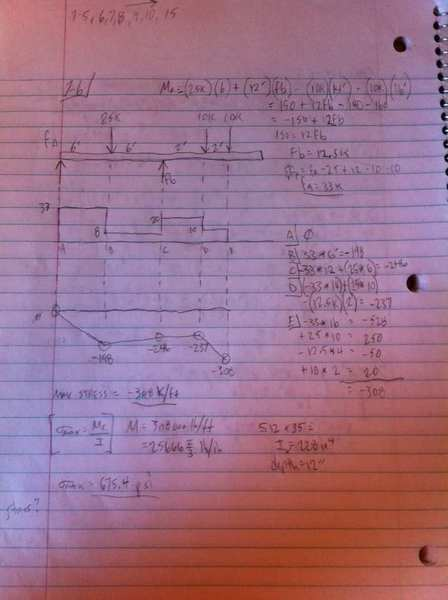# Is bending diagram correct?

## Homework Statement## Homework Equations

moment = force x distance

## The Attempt at a Solution

the book answer is different and doesn't show bending diagram. Can't tell what it should look like. Answer for maximum stress in book is way off:

m=60 k*ft, s=17.1 in^3, stress = m/s = 60000 lb*ft/38.1in^3 = 18900 psi

thanks

## Answers and Replies

AlephZero
Science Advisor
Homework Helper
It can't be right, because the BM diagram should be 0 at both ends if the beam is simply supported.

Your first line
M = -(25K)(6) + (12)(fb) .... etc
looks right. But on the next line you forgot the - sign and said
150 + 12 fb ...
not
-150 + 12 fb ...

After that, it looks like you did the right things, but the numbers are wrong.

As soon as you got the reaction force (incorrectly in this case) you should have checked it by concluding what the other reaction is AND taking moments about any point other than A, to check the correctness of your calculations so far. As you didn't do this, the rest is not valid. As your moment diagram is not correct, I would ask you to state which definition of bending moment you are using (there are two possible, each one derivable from the other).

Fine, but I checked the reactions with instructor and he said it was correct (Fb)

Calculation for Fa may be off by .5

Well, either your instructor is wrong or there is a mistake in your drawing. Incidentally, what is the length of the right hand unloaded length of the beam? So here's another check you can do. The centroid of the applied loads should coincide with the centroid of the reactions. (If they didn't coincide, what would be the implication?) Can you calculate those two positions? In your case they don't, even with a 0.5 variation. I asked you for the definition of bending moment for a very good reason, but you didn't answer my question. Why was that?